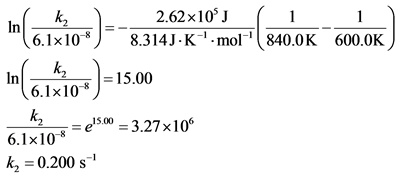# The following reaction has an activation energy of 262 kJ/mol

The following reaction has an activation energy of 262 kJ/mol.
C4H8(g) --> 2C2h4(g)

At 600.0 K the rate constant is 6.1× 10–8 s–1. What is the value of the rate constant at 840.0 K?
please explain in detail. this shits confusing.

Concepts and reason
Arrhenius equation is used to determine the rate constant at a different temperature.

The Arrhenius equation is as follows: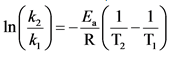Fundamentals
Activation energy is the minimum energy required to occur the reaction. In general, it is equal to the difference in energy between the transition state and the reactants.

Consider the reaction as follows: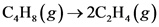The given data is as follows: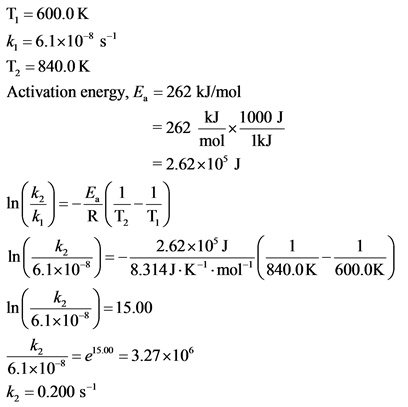Explanation: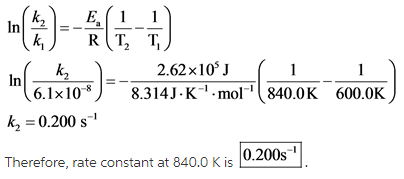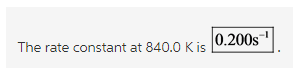Explanation:
All the values are substituted in the equation and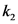is calculated as follows: